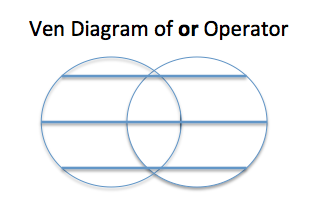# The Or Keyword In Python

## Overview:

• The, or keyword implements the logical OR operation in Python.
• In Mathematics the OR operator returns True, if at least one of the operands evaluate to True.
• In Electronics the OR Gate implements the logical OR and the input and output are described by the truth table given below.
• Remember in Python or operator does not return True or False. The, or operator in Python returns the first operand if it is True else the second operand.

## Truth table of the or Operator:

 Operand 1 Operand 2 Result True True True False True True True False True False False False

## Ven Diagram of the or operator:## Example 2:

 # Example python program using or operator def buy():     print("Buy order placed")   def sell():     print("Sell order placed")   indicator1 = 10 indicator2 = 1.5   if indicator1 >= 10 or indicator2 < 1:     buy()   else:     sell()